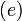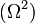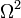# User:Tohline/AxisymmetricConfigurations/Storyline

(Difference between revisions)
 Revision as of 19:06, 5 July 2019 (view source)Tohline (Talk | contribs) (Created page with '__FORCETOC__ =(Initially) Axisymmetric Configurations=
…')← Older edit Current revision as of 15:08, 15 August 2019 (view source)Tohline (Talk | contribs) (→(Initially) Axisymmetric Configurations) (8 intermediate revisions not shown) Line 10: Line 10:
— Drawn from [https://ui.adsabs.harvard.edu/abs/1967ARA%26A...5..465L/abstract N. R. Lebovitz (1967)], ARAA, 5, 465 — Drawn from [https://ui.adsabs.harvard.edu/abs/1967ARA%26A...5..465L/abstract N. R. Lebovitz (1967)], ARAA, 5, 465 -
- We add a third category, namely, the nonlinear dynamical evolution of systems that are revealed via stability analyses to be unstable.
- + + Once you have learned how to construct spherically symmetric, equilibrium self-gravitating configurations from gases that obey a variety of different equations of state, it is natural to ask how those structures will be modified if they are rotating.  You might naturally ask, as well, how techniques that you have learned to use to examine the stability of each spherically symmetric, equilibrium configuration — principally, linear stability analyses and free-energy analyses — might be extended to permit you to examine the stability of rotating equilibrium structures.  As is suggested by the above quotation from [https://ui.adsabs.harvard.edu/abs/1967ARA%26A...5..465L/abstract N. R. Lebovitz (1967)], much of our discussion of axisymmetric configurations will be focused on an examination of published literature that addresses these two broad topics.  Then, pursuing a review of even broader scope, we will examine what has been learned over the past few decades regarding the dynamical evolution of — and the associated nonlinear development of (principally) nonaxisymmetric structure in — systems that have been revealed via stability analyses to be unstable. Line 19: Line 18: ==Storyline== ==Storyline== - Once you have learned how to construct spherically symmetric, equilibrium self-gravitating configurations from gases that obey a variety of different equations of state, it is natural to ask how those structures will be modified if they are rotating.  You might naturally ask, as well, how techniques that you have learned to use to examine the stability of each spherically symmetric, equilibrium configuration might be modified to permit you to examine the stability of rotating structures. + For more than 250 years the astrophysics (and mathematics) community has understood ''which'' set of equations must be solved simultaneously in order to build equilibrium models of rotating, self-gravitating gases.  As has been recounted by [https://onlinelibrary.wiley.com/doi/abs/10.1002/cpa.3160200203 Chandrasekhar (1967)], even Isaac Newton took a stab at estimating the degree to which the Earth's shape differs from that of a perfect sphere as a result of its rotation.  But, broadly speaking, this set of equations is difficult to solve without the aid of a computer.  So, with one notable exception, our understanding of the properties of rotating equilibrium configurations — let alone their relative stability — was not significantly advanced until the 1960s. + + The notable exception is the vast field of research on the equilibrium and stability of rotating, self-gravitating ''incompressible'' fluids, all of which stems from the book by [[User:Tohline/Apps/MaclaurinSpheroids/GoogleBooks#Excerpts_from_A_Treatise_of_Fluxions|Colin Maclaurin (1742)]] titled, ''A Treatise of Fluxions''.  In effect, [[User:Tohline/Apps/MaclaurinSpheroids#Maclaurin_Spheroids_.28axisymmetric_structure.29|Maclaurin showed]] that the gravitational potential of a uniform-density (polytropic index, n = 0) spheroid can be determined analytically and that detailed force balance can be achieved ''throughout'' an oblate-spheroidal configuration if, for a specified degree of flattening at the poles, the spheroid spins as a solid body with a uniquely specified frequency.  From this exceptionally insightful work, the equilibrium properties of a sequence of uniformly rotating, incompressible spheroids can be quantitatively enumerated. + + +
+ + "The Maclaurin sequence is a sequence of oblate spheroids along which the eccentricity [itex]~(e)[/itex] of the meridional sections increases from zero to one.  A feature of the sequence which caused considerable surprise, at the time it was discovered by D'Alembert, is that along the sequence the square [itex]~(\Omega^2)[/itex] of the angular velocity of rotation is not a parameter of unrestricted range; and that for each value of [itex]~\Omega^2[/itex], less than a certain determinate maximum, there are two permissible spheroidal figures of equilibrium.  It was indeed this last circumstance that led Jacobi first to suspect, and then to verify, that a sequence of genuine tri-axial ellipsoids of equilibrium branches off from the Maclaurin sequence." +
+ — Drawn from §2a of [https://ui.adsabs.harvard.edu/abs/1964ApNr....9..323C/abstract S. Chandrasekhar & N. R. Lebovitz (1964)], Astrophysica Norvegica, 9, 232 +

# (Initially) Axisymmetric Configurations

 "As a practical matter, discussions of the effect of rotation on self-gravitating fluid masses divide into two categories: the structure of steady-state configurations, and the oscillations and the stability of these configurations." — Drawn from N. R. Lebovitz (1967), ARAA, 5, 465

Once you have learned how to construct spherically symmetric, equilibrium self-gravitating configurations from gases that obey a variety of different equations of state, it is natural to ask how those structures will be modified if they are rotating. You might naturally ask, as well, how techniques that you have learned to use to examine the stability of each spherically symmetric, equilibrium configuration — principally, linear stability analyses and free-energy analyses — might be extended to permit you to examine the stability of rotating equilibrium structures. As is suggested by the above quotation from N. R. Lebovitz (1967), much of our discussion of axisymmetric configurations will be focused on an examination of published literature that addresses these two broad topics. Then, pursuing a review of even broader scope, we will examine what has been learned over the past few decades regarding the dynamical evolution of — and the associated nonlinear development of (principally) nonaxisymmetric structure in — systems that have been revealed via stability analyses to be unstable.

## Storyline

For more than 250 years the astrophysics (and mathematics) community has understood which set of equations must be solved simultaneously in order to build equilibrium models of rotating, self-gravitating gases. As has been recounted by Chandrasekhar (1967), even Isaac Newton took a stab at estimating the degree to which the Earth's shape differs from that of a perfect sphere as a result of its rotation. But, broadly speaking, this set of equations is difficult to solve without the aid of a computer. So, with one notable exception, our understanding of the properties of rotating equilibrium configurations — let alone their relative stability — was not significantly advanced until the 1960s.

The notable exception is the vast field of research on the equilibrium and stability of rotating, self-gravitating incompressible fluids, all of which stems from the book by Colin Maclaurin (1742) titled, A Treatise of Fluxions. In effect, Maclaurin showed that the gravitational potential of a uniform-density (polytropic index, n = 0) spheroid can be determined analytically and that detailed force balance can be achieved throughout an oblate-spheroidal configuration if, for a specified degree of flattening at the poles, the spheroid spins as a solid body with a uniquely specified frequency. From this exceptionally insightful work, the equilibrium properties of a sequence of uniformly rotating, incompressible spheroids can be quantitatively enumerated.

 "The Maclaurin sequence is a sequence of oblate spheroids along which the eccentricity$~(e)$ of the meridional sections increases from zero to one. A feature of the sequence which caused considerable surprise, at the time it was discovered by D'Alembert, is that along the sequence the square$~(\Omega^2)$ of the angular velocity of rotation is not a parameter of unrestricted range; and that for each value of$~\Omega^2$, less than a certain determinate maximum, there are two permissible spheroidal figures of equilibrium. It was indeed this last circumstance that led Jacobi first to suspect, and then to verify, that a sequence of genuine tri-axial ellipsoids of equilibrium branches off from the Maclaurin sequence." — Drawn from §2a of S. Chandrasekhar & N. R. Lebovitz (1964), Astrophysica Norvegica, 9, 232